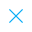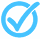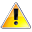Call Support +91-85588-96644

Keep me logged in
You can't leave Captcha Code empty
By submitting this form, you agree to the Terms & Privacy Policy.
ORTests given# Linear Equation in One Variable - 1 (Class - IX) By TCY All tests

Questions

Time

Highest
score

Level

## ENGLISH

Language

Topics Covered:Application of Linear EquationsLinear EquationFinding Solution of a Given Linear EquationLinear Equations in One and Two VariablesLinear Equations with One and Two VariablesPolynomialsSimple EquationsSolving equationsEquations